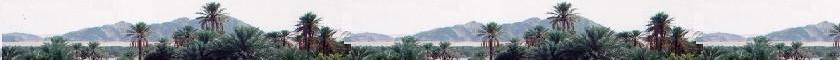Quantum Mechanics

Schrodinger equation

Quantum Mechanics

Propagators : Pg

Quantum Simple Harmonic
Oscillator QSHO

Quantum Mechanics
Simulation With GNU Octave

© The scientific sentence. 2010

# Quantum harmonic oscillator Ladder Operators

### 1.Hamiltonian of the One-dimensional SHO

```Let the particle of mass m represents an harmonic oscillator.
Its Hamiltonian is:

H = p2/2m + mω2x2/2

Where x is position operator and p is the momentum operator.
They are given by:

x = x
p = - i ℏ∂/∂x

To find the energy eigenstates and their corresponding energy
levels, we must solve the time-independent Schr�dinger equation
H|ψ> = E|ψ>.

```

### 2. Ladder operator method

```The "ladder operator" method is used to find the energy
eigenvalues without directly solving the differential equation.
We define two operators a and its adjoint a+ as follows:

a  = [mω/2ℏ]1/2 [x + (i/mω)p]
a+ = [mω/2ℏ]1/2 [x - (i/mω)p]

Let's define the product:

N = a+a

N = [mω/2ℏ] [x2 + (i/mω)xp - (i/mω)px + (1/mω)2p2]
= [mω/2ℏ] [x2 + (i/mω)[x,p] + (1/mω)2p2]
[x,p]= iℏ, so:
N = [mω/2ℏ] [x2 - (ℏ/mω) + (1/mω)2p2] =
N = a+a = (mω/2ℏ) x2 + (1/2ℏmω) p2 - 1/2

Now, Let's write:
(N + 1/2) ℏ ω  = (mω2/2)x2 + (1/2m)p2] = H

Therefore, the Hamiltonian of the quantum harmonic
oscillator can be written in terms of thw ladder
operators:

H = (N + 1/2)ℏω

We have also:
(aa+ - 1/2)ℏω = H  = (N + 1/2)ℏω, so:

(aa+)ℏω = (N + 1)ℏω, then: aa+ = N + 1

aa+ = N + 1

Commutators:
1.
[a, a+] = aa+ - a+a =  - N + N + 1 = 1

2.
[N,a+] = Na+ - a+N = a+aa+ - a+a+a =
a+aa+ - a+(aa+ - 1) = a+aa+ - a+aa+ + a+ = a+

3.
[N,a] = Na - aN = a+aa - aa+a = (a+a - aa+)a =
[a+,a]a = - a

[a, a+] = 1
[N,a+]  = a+
[N,a]  = - a

Let's write the eigenstate for the quantum harmonic
oscillator ψn(x)= |n>. The number operator N satisfy the
equation:
N|n> = n |n>

Na+|n> = (a+N + [N,a+])|n> = (a+N + a+)|n> =
a+(N + 1)|n> = a+(n + 1)|n> = (n + 1) a+|n>

Similarly,

Na|n> = (aN + [N,a])|n>: = (aN - a)|n> = (n - 1)a|n>

a+ is a raising operator
Na+|n>  = (n + 1) a+|n>
a is a lowering operator
Na|n> = (n - 1)a|n>

If the smallest eigen number n is 0 (ground state), then a|0> = 0
( we cannot lower less than 0).

Therefore:
H|0> = (ℏω/2)|0>

For the excited state

H|n> = En = (ℏω (n + 1/2)|n>.
Its corresponding eigenstate is:
|n> = [(a+)n/(n!)1/2]|o>

This expression comes from the following proof:
We force  a+ to satisfy:
a+|n> = C |n+1>, so
<n|aa+|n> = |C|2 <n|n+1>
n + 1 , then:
C = (n+1)1/2, therefore:
a+|n> = (n+1)1/2|n+1>

Thus:
By n-1 instead of n in a+|n> = (n+1)1/2|n+1>, we get:
|n+1> = (n+1)- 1/2 a+|n> and
|n> = n- 1/2 a+|n-1>

By n-2 instead of n in a+|n> = (n+1)1/2|n+1>, we get:

a+|n-2> = (n-1)1/2|n-1>
so |n-1> = a+|n-2>/(n-1)1/2
By substitution |n-1> in |n> = n- 1/2 a+|n-1>, we get:
|n> = n- 1/2 a+ a+|n-2>/(n-1)1/2; =  n- 1/2 (n-1)- 1/2a+2|n-2>

... and so on ...
=
|n> =  (n!)- 1/2 a+n|0>

Finally, without solving the equation of Schrodinger,
we have get the expressions of the eigenvalue and the
eigenstate for an quantum harmonic oscillator:

En = (ℏω (n + 1/2)
|n> =  (n!)- 1/2 (a+)n|0>

```

 chimie labs | Physics and Measurements | Probability & Statistics | Combinatorics - Probability | Chimie | Optics | contact |